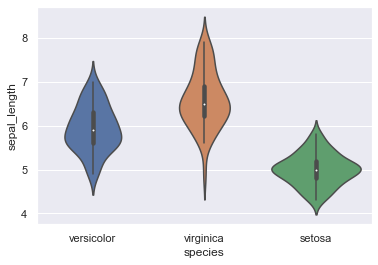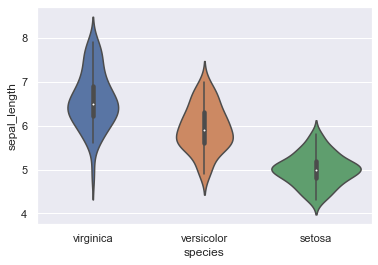# Manage the order of groups in seaborn violinplot

One could decide to change the order in which groups appear in a given plot, wether it be for the sake of clarity or other reasons. This can be performed with the 'order' parameter, either by specifying directly the order of the groups through a list, or by generating an ordered list based on the groups corresponding mean for example.

## Directly specyfing a list

Thanks to the 'order' parameter of the violinplot function, you can set the order in which you expect the distributions to appear on the figure.

``````# libraries & dataset
import seaborn as sns
import matplotlib.pyplot as plt
# set a grey background (use sns.set_theme() if seaborn version 0.11.0 or above)
sns.set(style="darkgrid")

# specifying the group list as 'order' parameter and plotting
sns.violinplot(x='species', y='sepal_length', data=df, order=[ "versicolor", "virginica", "setosa"])
plt.show()``````## Ordering by decreasing median

Instead of creating the list 'by hand' with various categories, you can also use the power of pandas operations (groupby, median or mean) in order to create a ranked list, which we stored in 'my_order' variable in the following example.

``````# libraries & dataset
import seaborn as sns
import matplotlib.pyplot as plt
# set a grey background (use sns.set_theme() if seaborn version 0.11.0 or above)
sns.set(style="darkgrid")

# Using pandas methods and slicing to determine the order by decreasing median
my_order = df.groupby(by=["species"])["sepal_length"].median().iloc[::-1].index

# Specifying the 'order' parameter with my_order and plotting
sns.violinplot(x='species', y='sepal_length', data=df, order=my_order)
plt.show()``````## Contact & Edit

👋 This document is a work by Yan Holtz. Any feedback is highly encouraged. You can fill an issue on Github, drop me a message onTwitter, or send an email pasting `yan.holtz.data` with `gmail.com`.